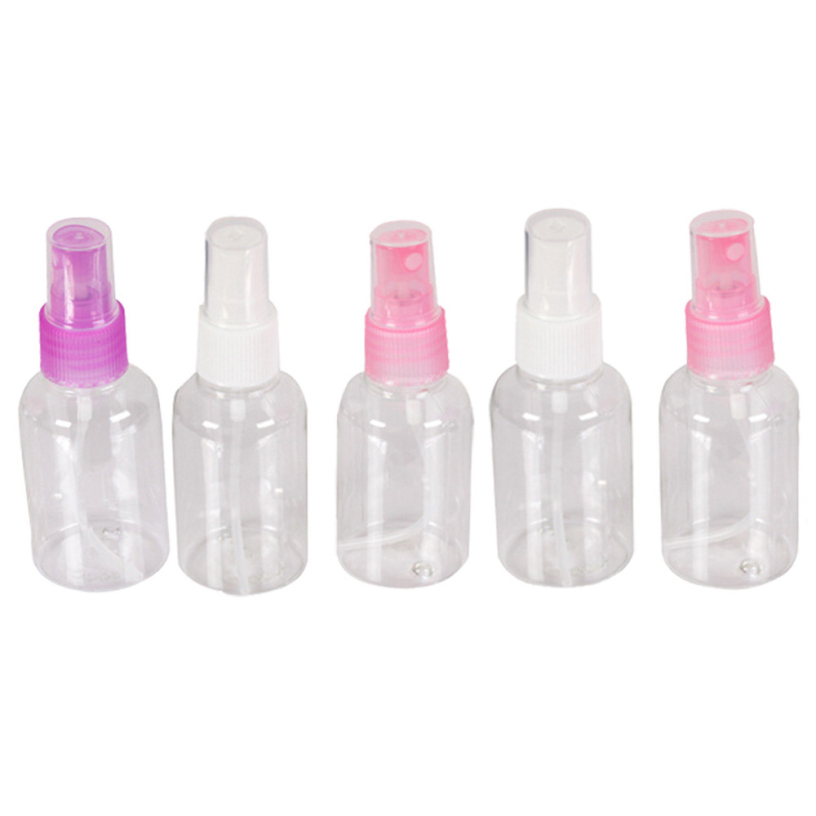#1 P C S R a n d o m C o l o r T r a v e l T r a n s p a r e n t P l a s t i c P e r f u m e A t o m i z e r S m a l l M I n i E m p t y S p r a y R e f i l l a b l e B o t t l e

Updated Friday 25-Jan-2019## 1 P C S R a n d o m C o l o r T r a v e l T r a n s p a r e n t P l a s t i c P e r f u m e A t o m i z e r S m a l l M I n i E m p t y S p r a y R e f i l l a b l e B o t t l e 11

THB 35.96

THB 35.46 Mon 04-Mar-2019

More details at ezbuy.co.th